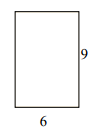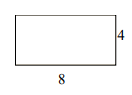# Areas of rectangles with the same perimeter

In this lesson we find areas of rectangles with same perimeters as that of given rectangles.

We are given some rectangles with certain dimensions. We find their perimeters. We are now required to find rectangles with same perimeters as those of given rectangles but with different dimensions and then find their areas also.

The rectangle below has the dimensions 9×6. Create a rectangle with the same perimeter, but a different area.### Solution

Step 1:

Perimeter of given rectangle = 2(l + w) = 2(9 + 6) = 30; Area = l × w = 9 × 6 = 54 square units

Step 2:

One possible rectangle with same perimeter has dimensions 8×7; Its perimeter = 2(7 + 8) = 30

Its area that is different = 7 × 8 = 56 square units

The rectangle below has the dimensions 8 × 4. Create a rectangle with the same perimeter, but a different area.### Solution

Step 1:

Perimeter of given rectangle = 2(l + w) = 2(8 + 4) = 24; Area = l × w = 8 × 4 = 32 square units

Step 2:

One possible rectangle with same perimeter has dimensions 7 × 5; Its perimeter = 2(7 + 5) = 24

Its area that is different = 7 × 5 = 35 square units